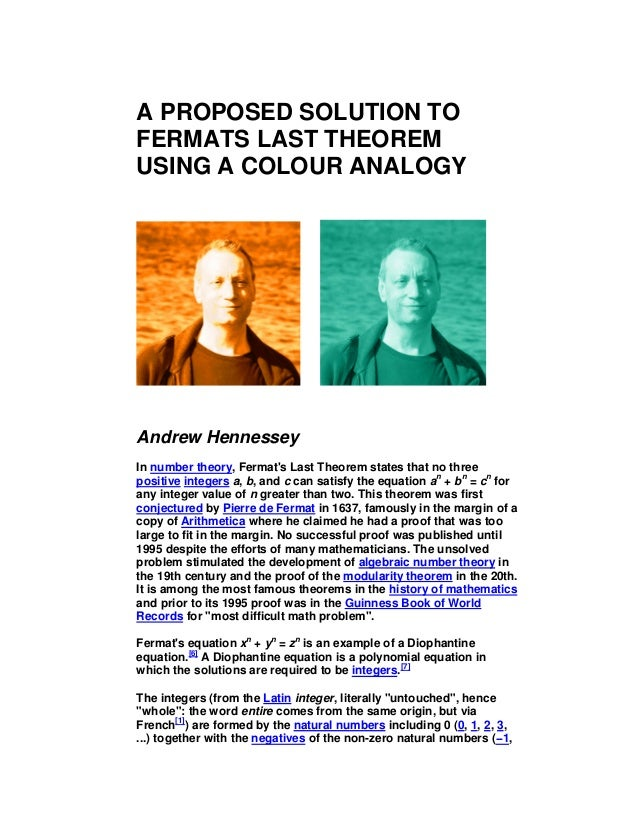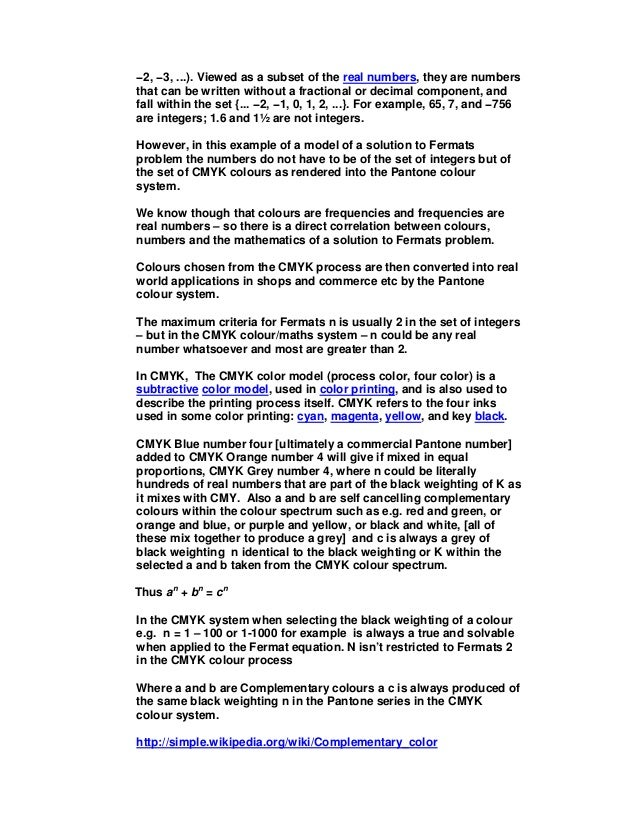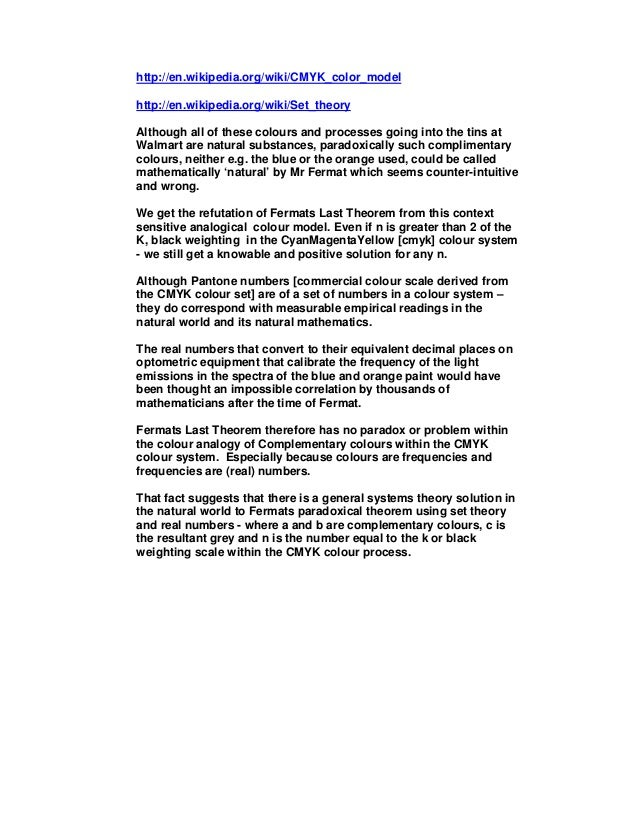Successfully reported this slideshow.
We use your LinkedIn profile and activity data to personalize ads and to show you more relevant ads. You can change your ad preferences anytime.Upcoming SlideShare
Loading in …5
×

# A solution to Fermats last theorem using a colour analogy

I know - Fermats Last Theorem - who can possibly solve something as mind bendingly difficult as this ... tens of thousands of eggheads have tried and failed ... etc etc

Don't worry I'm not looking for a Nobel Prize - but you never know - if tens of thousands of top class mathematicians couldn't get a job mixing paint in Walmart - there must be possibilities here somehow ...

• Full Name
Comment goes here.

Are you sure you want to Yes No
Your message goes here• Be the first to comment

• Be the first to like this

### A solution to Fermats last theorem using a colour analogy

1. 1. A PROPOSED SOLUTION TO FERMATS LAST THEOREM USING A COLOUR ANALOGY Andrew Hennessey In number theory, Fermat's Last Theorem states that no three positive integers a, b, and c can satisfy the equation an + bn = cn for any integer value of n greater than two. This theorem was first conjectured by Pierre de Fermat in 1637, famously in the margin of a copy of Arithmetica where he claimed he had a proof that was too large to fit in the margin. No successful proof was published until 1995 despite the efforts of many mathematicians. The unsolved problem stimulated the development of algebraic number theory in the 19th century and the proof of the modularity theorem in the 20th. It is among the most famous theorems in the history of mathematics and prior to its 1995 proof was in the Guinness Book of World Records for "most difficult math problem". Fermat's equation xn + yn = zn is an example of a Diophantine equation. A Diophantine equation is a polynomial equation in which the solutions are required to be integers. The integers (from the Latin integer, literally "untouched", hence "whole": the word entire comes from the same origin, but via French ) are formed by the natural numbers including 0 (0, 1, 2, 3, ...) together with the negatives of the non-zero natural numbers (−1,
2. 2. −2, −3, ...). Viewed as a subset of the real numbers, they are numbers that can be written without a fractional or decimal component, and fall within the set {... −2, −1, 0, 1, 2, ...}. For example, 65, 7, and −756 are integers; 1.6 and 1½ are not integers. However, in this example of a model of a solution to Fermats problem the numbers do not have to be of the set of integers but of the set of CMYK colours as rendered into the Pantone colour system. We know though that colours are frequencies and frequencies are real numbers – so there is a direct correlation between colours, numbers and the mathematics of a solution to Fermats problem. Colours chosen from the CMYK process are then converted into real world applications in shops and commerce etc by the Pantone colour system. The maximum criteria for Fermats n is usually 2 in the set of integers – but in the CMYK colour/maths system – n could be any real number whatsoever and most are greater than 2. In CMYK, The CMYK color model (process color, four color) is a subtractive color model, used in color printing, and is also used to describe the printing process itself. CMYK refers to the four inks used in some color printing: cyan, magenta, yellow, and key black. CMYK Blue number four [ultimately a commercial Pantone number] added to CMYK Orange number 4 will give if mixed in equal proportions, CMYK Grey number 4, where n could be literally hundreds of real numbers that are part of the black weighting of K as it mixes with CMY. Also a and b are self cancelling complementary colours within the colour spectrum such as e.g. red and green, or orange and blue, or purple and yellow, or black and white, [all of these mix together to produce a grey] and c is always a grey of black weighting n identical to the black weighting or K within the selected a and b taken from the CMYK colour spectrum. Thus an + bn = cn In the CMYK system when selecting the black weighting of a colour e.g. n = 1 – 100 or 1-1000 for example is always a true and solvable when applied to the Fermat equation. N isn’t restricted to Fermats 2 in the CMYK colour process Where a and b are Complementary colours a c is always produced of the same black weighting n in the Pantone series in the CMYK colour system. http://simple.wikipedia.org/wiki/Complementary_color
3. 3. http://en.wikipedia.org/wiki/CMYK_color_model http://en.wikipedia.org/wiki/Set_theory Although all of these colours and processes going into the tins at Walmart are natural substances, paradoxically such complimentary colours, neither e.g. the blue or the orange used, could be called mathematically ‘natural’ by Mr Fermat which seems counter-intuitive and wrong. We get the refutation of Fermats Last Theorem from this context sensitive analogical colour model. Even if n is greater than 2 of the K, black weighting in the CyanMagentaYellow [cmyk] colour system - we still get a knowable and positive solution for any n. Although Pantone numbers [commercial colour scale derived from the CMYK colour set] are of a set of numbers in a colour system – they do correspond with measurable empirical readings in the natural world and its natural mathematics. The real numbers that convert to their equivalent decimal places on optometric equipment that calibrate the frequency of the light emissions in the spectra of the blue and orange paint would have been thought an impossible correlation by thousands of mathematicians after the time of Fermat. Fermats Last Theorem therefore has no paradox or problem within the colour analogy of Complementary colours within the CMYK colour system. Especially because colours are frequencies and frequencies are (real) numbers. That fact suggests that there is a general systems theory solution in the natural world to Fermats paradoxical theorem using set theory and real numbers - where a and b are complementary colours, c is the resultant grey and n is the number equal to the k or black weighting scale within the CMYK colour process.